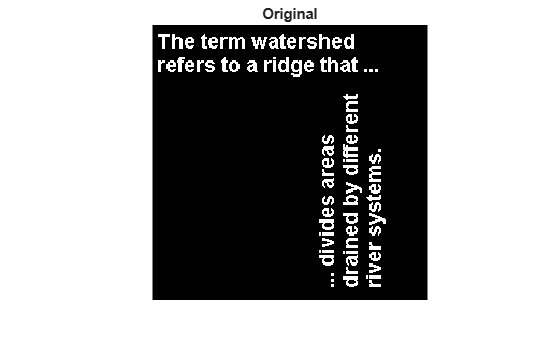# imdilate

## 语法

``J = imdilate(I,SE)``
``J = imdilate(I,nhood)``
``J = imdilate(___,packopt)``
``J = imdilate(___,shape)``

## 说明

``J = imdilate(I,SE)` 使用结构元素 `SE` 膨胀灰度、二值或压缩二值图像 `I`。`
``J = imdilate(I,nhood)` 对图像 `I` 执行扩张运算，其中 `nhood` 是由 `0` 和 `1` 组成的矩阵，用于指定结构元素邻域。此语法等效于 `imdilate(I,strel(nhood))`。`
``J = imdilate(___,packopt)` 指定 `I` 是否为压缩二值图像。`

``J = imdilate(___,shape)` 指定输出图像的大小。`

## 示例

`BW = imread('text.png');`

`se = strel('line',11,90);`

```BW2 = imdilate(BW,se); imshow(BW), title('Original')````figure, imshow(BW2), title('Dilated')``originalI = imread('cameraman.tif');`

`se = offsetstrel('ball',5,5);`

`dilatedI = imdilate(originalI,se);`

`imshowpair(originalI,dilatedI,'montage')``se1 = strel('line',3,0)`
```se1 = strel is a line shaped structuring element with properties: Neighborhood: [1 1 1] Dimensionality: 2 ```
`se2 = strel('line',3,90)`
```se2 = strel is a line shaped structuring element with properties: Neighborhood: [3x1 logical] Dimensionality: 2 ```

`composition = imdilate(1,[se1 se2],'full')`
```composition = 3×3 1 1 1 1 1 1 1 1 1 ```

```BW = false(100,100,100); BW(25,25,25) = true; BW(75,75,75) = true;```

```se = strel('sphere',25); dilatedBW = imdilate(BW,se);```

```figure isosurface(dilatedBW, 0.5)```## 输入参数

`imdilate` 可对除数据类型为 `logical` 的图像之外的所有图像执行灰度膨胀。在这种情况下，结构元素必须为平面结构，并且 `imdilate` 执行二值膨胀。

`'notpacked'`

`I` 被视为普通数组。

`'ispacked'`

`I` 被视为由 `bwpack` 生成的压缩二值图像。`I` 必须为二维 `uint32` 数组，`SE` 必须为平面二维结构元素。`shape` 的值必须为 `'same'`

`'same'`

`'full'`

## 详细信息

### 二值膨胀

`$A\oplus B=\left\{z|{\left(\stackrel{^}{B}\right)}_{z}\cap A\ne \varnothing \right\},$`

### 灰度膨胀

`$\left(A\oplus B\right)\left(x,y\right)=\mathrm{max}\left\{A\left(x-{x}^{\prime },y-{y}^{\prime }\right)+B\left({x}^{\prime },{y}^{\prime }\right)|\left({x}^{\prime },{y}^{\prime }\right)\in {D}_{B}\right\},$`

`$\left(A\oplus B\right)\left(x,y\right)=\mathrm{max}\left\{A\left(x-{x}^{\prime },y-{y}^{\prime }\right)|\left({x}^{\prime },{y}^{\prime }\right)\in {D}_{B}\right\}.$`

## 提示

• 如果图像 `I` 的维度大于结构元素的维度，则 `imdilate` 函数会将相同的形态学膨胀应用于较高维度上的所有平面。

您可以使用此行为对 RGB 图像执行形态学膨胀。为 RGB 图像指定二维结构元素，以便对每个颜色通道分别执行运算。

• 当指定结构元素邻域时，`imdilate` 通过 `floor((size(nhood)+1)/2)` 确定 `nhood` 的中心元素。

• `imdilate` 自动利用结构元素对象的分解（如果存在分解）。此外，当使用具有分解的结构元素对象执行二值膨胀时，`imdilate` 会自动使用二值图像压缩来加速膨胀

 Gonzalez, Rafael C., Richard E. Woods, and Steven L. Eddins. Digital Image Processing Using MATLAB. Third edition. Knoxville: Gatesmark Publishing, 2020.

 Haralick, Robert M., and Linda G. Shapiro. Computer and Robot Vision. 1st ed. USA: Addison-Wesley Longman Publishing Co., Inc., 1992, pp. 158-205.

 Boomgaard, Rein van den, and Richard van Balen. “Methods for Fast Morphological Image Transforms Using Bitmapped Binary Images.” CVGIP: Graphical Models and Image Processing 54, no. 3 (May 1, 1992): 252–58. https://doi.org/10.1016/1049-9652(92)90055-3.